```

```
Wholeness and the Implicate Order
```
```
The Crystallization Process and the Implicate Order
(Part Seventeen)

```

```
We  continue our investigation concerning the generation of group elements of the Plane Groups.
```
```
The Plane Group P6mmFigure 1.  When motifs, having point symmetry 6mm (i.e. having a 6-fold rotation axis and two types of mirror lines), are inserted into a (primitive) hexagonal net, a pattern of repeated motifs will emerge that represents Plane Group P6mm.
Also here the unit mesh is rhomb-shaped with angles of 600 and 1200.
In the present representation each (composed) motif consists of six motif units, together making up a composed motif with
6mm  symmetry. The pattern must be conceived to be extended indefinitely in two-dimensional space.

```
```
The total symmetry content of Plane Group P6mm is depicted in Figure 2.Figure 2. Total symmetry content of the Plane Group P6mm.
All rotation axes are perpendicular to the plane of the drawing.
6-fold rotation axes are indicated by small blue solid hexagons.
3-fold rotation axes are indicated by small blue solid triangles.
2-fold rotation axes are indicated by small blue solid ellipses.
Glide lines are indicated by red dashed lines.
Mirror lines are indicated by solid lines (red and black).
(One should not worry about the small discrepancies at some places in the drawing.)

```
```
Figure 1 gives an accurate representation of the Plane Group P6mm. All the symmetries of the group are present in the image of that Figure. So for analytic purposes (analytic group theoretic approach) the representation is appropriate.
However, for a synthetic group theoretical approach -- involving the generation of the group -- the figure is, in a way, not detailed enough. In it we see a composed motif consisting of six motif units :But we also see that each motif unit itself is still composed, namely (composed) out of two symmetrical halves. So such a symmetrical motif unit cannot represent a group element, it represents already two group elements. The genuine motif unit, representing a group element, is therefore half the motif unit as set initially. This means that each whole composed motif, as depicted above (and in Figure 1), consists not of six, but of twelve genuine motif units. And these newly conceived motif units are indeed asymmetric, that is to say they cannot be further divided anymore. And they indeed can represent the group elements :To highlight these genuine motif units more clearly, we can use colors, provided we do not interpret the difference in color to represent an asymmetry :  one motif unit (red or blue) is asymmetric. Two of them together (one red and one blue) make up a symmetric entity (representing not a group element but a subgroup of the full group), and twelve of them (six red, six blue) make up the full composed hexagonal motif :The next Figure has such motifs be placed in a hexagonal net (point lattice) :Figure 3.  A representation of a  P6mm  pattern, in terms of genuine basic motif units (red and blue). Here the difference in color should not be interpreted as asymmetry, it only serves to indicate the basic motif units (making up the hexagonal composed motifs). Each such composed motif is supposed to have  6mm  symmetry, i.e. it has  D6  structure (not  C6  structure). It has therefore six rotations and six reflections. Each (genuine) motif unit (either red or blue) represents a group element of the Plane Group  P6mm .

```
```
In order to generate the  P6mm  pattern, which means to generate the Plane Group P6mm, which in turn means to generate all group elements, we choose one motif unit to be the initial motif unit and as such to represent the identity element, denoted  1 ,  and three others to represent generator elements :

A generator element  m ,  resulting from a reflection of the initial motif unit in a mirror line  m .
A generator element  p ,  resulting from a 600 anticlockwise rotation of the initial motif unit about the point  R  (See next Figure).
A generator element  t ,  resulting from a horizontal translation  t  of the initial motif unit.Figure 4.  Three generators,  m,  p  and  t ,  represented by motif units, and one initial motif unit, representing the identity element, are chosen. The motif unit  p  results from a 600 anticlockwise rotation of the initial element  1  about the point  R .  The motif unit  t  results from a horizontal shift of the initial motif unit to the right.

```
```
For clarity we enlarge the region around the point R :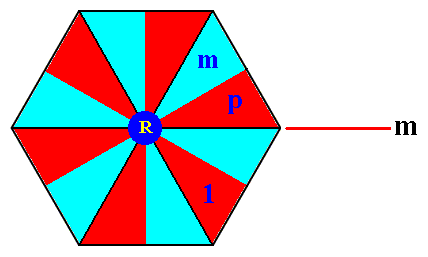Figure 5.  The composed motif (enlarged) from the  P6mm  pattern of the previous Figure at the point  R . It consists of twelve true motif units (red and blue), representing group elements. The difference in color should (here) not be interpreted as representing asymmetry.
The motif units
1 ,  m,  p  and the mirror line  m  are indicated.

```
```
The other motif units of the composed motif of Figure 5 are then automatically implied :Figure 6.  The composed motif and its twelve constituent basic motif units, at the point  R  of Figure 4.  These basic motif units represent the group elements  1, m, p, mp, mp2, mp3, mp4, mp5, p2, p3, p4  and  p5 .  They form the subgroup  D6  of the group  P6mm .  The elements  1, p,  p2, p3, p4, p5  also form a subgroup (of the group  P6mm  as well as of the subgroup  D6 ) with structure C6 .

```
```
In order to further generate the pattern (and with it the group elements) we can subject the elements of the subgroup D6 (Figure 6) to a translation  t  resulting in 12 new group elements (represented by 12 basic motif units). A few of these new elements are indicated in the next Figure.Figure 7.  Some new group elements resulting from the translation  t  are indicated. Also some points, bearing composed motifs, of the lattice are indicated (R , Q , U, W).

```
```
The next Figure shows the composed motif next to the right of the one at point R, with the group elements (represented by basic motif units) indicated, as they were produced by the translation  t  with respect to the composed motif at the point R.Figure 8.  The composed motif next to the right of the one at the point R .

```
```
The red motif units in Figure 8  together form the left coset of the subgroup {1, p  p2, p3, p4, p5 } (with structure C6 ) (See Figure 6) by the element  t .
All the twelve elements (red and blue in Figure 8 ) together form the left coset of the D6 subgroup (See Figure 6) by the element  t  (For the group theoretic concept  c o s e t  see our exposition of Group Theory in  Second Part of Website .  In the present context it is not of direct importance).

Next we're going to generate the composed motif at the point Q in Figure 7. It can be obtained by rotating the previously generated composed motif (the motif next to the one at point R) 3000 anticlockwise (or, which is the same, 600 clockwise) about the point R, which means that we subject all elements of the previously generated composed motif to the action of  p5 .
The next Figure gives this new composed motif. Inside the image we have left the notations for the elements as they were in the motif next to R, while the identities of the newly generated group elements are given at the perimeter of the image :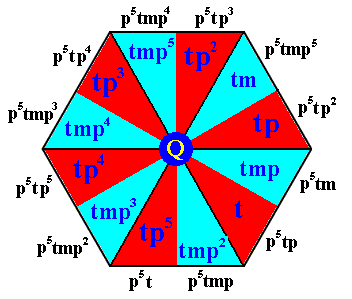Figure 9.  The newly generated composed motif at lattice point  Q  (as indicated in Figure 7). The names of the new elements are given at the perimeter of the image (i.e. outside the image).

```
```
All the elements in Figure 9  (red and blue) together form the left coset of the D6 subgroup (See Figure 6) by the element  p5t .

The third row (Figure 7) of composed motifs can now be completed by means of applying to this lastly obtained composed motif the translations  . . . t-3, t-2, t-1, t,  t2, t3 . . . . And along the same lines we can complete row 2 of the pattern.

In order to reach the fourth row we first determine the composed motif at the point U by applying   t2  ( =  2 times applying the translation  t ) to the elements of the composed motif at the point R :and then rotate it 3000 anticlockwise about the point R, i.e. applying  p5  :Figure 10.  The composed motif at the point  W  (Figure 7) is completed, by applying  p5  to the composed motif at the point  U .  The original element notations as they were at point U have been put inside the image, while at its perimeter we have put the notations of the newly generated elements of the composed motif at the point W.

```
```
The six red motif units of the above Figure (Figure 10) together form the left coset of the subgroup {1, p,  p2, p3, p4, p5} (with structure C6 ) by the element  p5t2 .
This fourth row can now be completed by means of translations.
It is clear that by using the rotations and translations we can generate in principle the whole group ( The generator  m, i.e. the reflection in the line  m ,  was needed to obtain a reflected motif unit, which then was multiplied by the rotations and translations, and these latter two transformations can never produce a reflected motif unit).
```

```
The same P6mm pattern can be generated by an augmented motif unit, which could be, for instance, two symmetrically related basic motif units :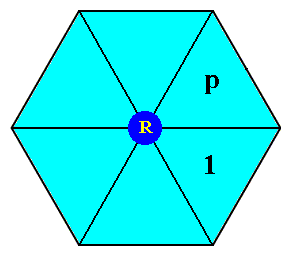Figure 11.  Composed motif (of the  P6mm  pattern) consisting of six symmetric motif units. Two of them are provided with a notation. Each such motif unit in fact consists of two symmetrically related basic motif units. These non-basic motif units cannot represent group elements. The one denoted by  1  is in fact the subgroup  {1, mp}  (in the notation above [See Figure 6 ], referring to basic motif units), which has the structure of  C2  ( The element  mp  is of period 2, which can easily be verified in Figure 6 ,  and a set consisting of the identity element and a period 2 element is a group of  C2  structure).

```
```
We will now generate the P6mm pattern by means of the non-basic motif units established in Figure 11.  We will use the same notation as we did with respect to the basic motif units. Because we now use symmetrical motif units (which, as has been said, consists of two symmetrically related basic motif units), we do not need the generator  m  anymore. We can do it with the generators  p (rotation) and  t  (translation). So now to begin with we can generate the other elements of the composed motif (representing the subgroup D6 ) :Figure 12.  By the generator  p  the remaining elements of the subgroup  D6  are generated :  Given  1  (initial element) and  p  (generator),  the element  p2  results after two times applying  p  to the initial element. The element  p3  results from three times applying  p ,  etc.,  while  p6 = 1 ,  where  p  is an anticlockwise rotation of 600 about the point  R .

```
```
The next Figure summarizes the two chosen generators, as represented by non-basic motif units (augmented motif units), that can generate the whole group P6mm :
The element (augmented motif unit)  p ,  resulting (as just established) from the element  1  (which is chosen to represent the identity element), by an anticlockwise rotation of 600 about the point  R .
The element (augmented motif unit)  t  resulting from element  1  in virtue of the translation  t .
We must realize that, although speaking about "elements", they are not true elements of the group, but subgroups or cosets.
If we were to insist that they are true group elements, then we would not in fact have to do with the group P6mm anymore, but with the group  P6  instead, i.e. with a subgroup of P6mm, because repeated rotation (there where p6 = 1) and translation of some initial entity results in the group structure of P6 (See Part XVI, where P6 is indeed generated from a rotation and a translation).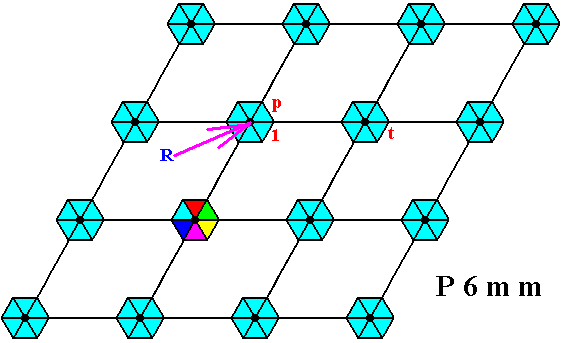Figure 13.  Two chosen generators,  p  and  t  for the  P6mm  pattern.
In one of the composed motifs (which are of course all identical) its composition out of six (augmented) motif units is indicated by colors.

```
```Figure 14.  Generation of the group  P6mm  by the generators  p  and  t .  See also next Figures.

```
```
The next Figures explain some of the generative relations between some group 'elements' (augmented motif units) established by applying the generator  p  five times to a particular (already generated) augmented motif unit. Five times applying  p  means applying  p5 ,  which in turn means an anticlockwise rotation of  5 x 60 = 3000 about the point  R  (as indicated in Figure 13). In the ensuing Figures augmented motif units and their images under  p5  are indicated by coloring.Figure 15.  Image  p5t2  -- under (the action of)  p5  -- of the augmented motif unit representing the group 'element'  t2 .
The action of
p5  is indicated by the circular arrow.

```
```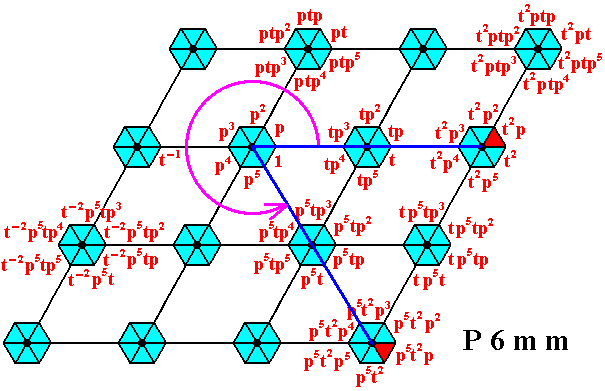Figure 16.  Image  p5t2p  -- under (the action of)  p5  -- of the augmented motif unit representing the group 'element'  t2p .
The action of
p5  is indicated by the circular arrow.

```
```Figure 17.  Image  p5t2p2  -- under (the action of)  p5  -- of the augmented motif unit representing the group 'element'  t2p2 .
The action of
p5  is indicated by the circular arrow.

```
```Figure 18.  Image  p5t2p3  -- under (the action of)  p5  -- of the augmented motif unit representing the group 'element'  t2p3 .
The action of
p5  is indicated by the circular arrow.

```
```Figure 19.  Image  p5t2p4  -- under (the action of)  p5  -- of the augmented motif unit representing the group 'element'  t2p4 .
The action of
p5  is indicated by the circular arrow.

```
```Figure 20.  Image  p5t2p5  -- under (the action of)  p5  -- of the augmented motif unit representing the group 'element'  t2p5 .
The action of
p5  is indicated by the circular arrow.

```

```
Next we will again generate our group  P6mm ,  but now letting the group elements not only be represented by the basic units of the motifs s.str. -- as in Figure 7 -- but also by their proper surroundings (background).
In order to find these surroundings as areas that -- with their content (basic unit of motif s.str.) -- can legitimately represent true group elements of the group P6mm, we must first determine an appropriate choice of motif s.l. ,  which can then be partitioned into the desired areas. See next Figure.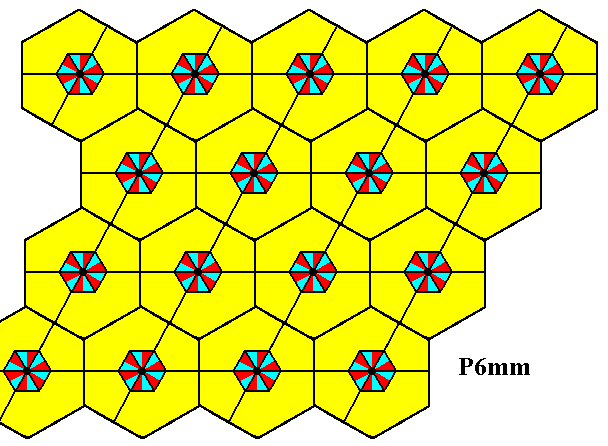Figure 21.  Choice of motifs s.l.  (yellow hexagons) of the  P6mm  pattern of Figure 3.
Each such motif s.l. consists of one motif s.str. PLUS corresponding background.

```
```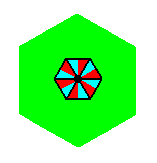Figure 22.  Motif s.l.  isolated (background differently colored than in the previous Figure).

```
```
The next Figure shows the partition of the motifs s.l. in such a way that each motif s.l. is divided into twelve equally sized and shaped areas. Each such area contains one basic unit of the motif s.str. PLUS corresponding background, and as such can represent a group element.Figure 23.  Partition of the motifs s.l. (as given in Figure 21 and 22), and with it (a partition) of the  P6mm  pattern, resulting in areas (green and yellow right-angled triangles) that can (together with their content) represent group elements of the Plane Group P6mm.

```
```Figure 24.  One partitioned motif s.l.  isolated. The areas A, B, C, D, E, F, G, H, I, J, K, L, including their content, which consists of one basic unit of the motif s.str., represent group elements.

```
```Figure 25.  One area, representing one group element, isolated.

```
```
The next Figure indicates the initial element and the three generators  p, m and  t .Figure 26.  The  P6mm  pattern partitioned into areas that represent group elements (as was already done in Figure 23). Four such group elements are explicitly indicated :
The initial group element
1  (identity element of the group).
The generator element
p ,  which is an anticlockwise rotation of 600 about the point  R .
The generator element
m ,  which is a reflection in the line  m .
The generator element
t , which is a horizontal translation.

```

```
In the next document we will generate the other group elements of our  P6mm  pattern.

e-mail :```
```
To continue click HERE for further study of the totally dynamic and holistic nature of Reality.
```
```
back to homepage

****************